• 8
•
•
•
•
•
•
•
8
Shares

# APPLICATION OF GAUSS’S LAW

So far we have learnt about the gauss’s law in detail. In this article, we are going to talk about the application of gauss’s law. So keep reading till end.

We know that gauss law is a law which relates the distribution of electric charge to the resulting electric field. Means, we can find the value of electric field by using gauss’s law.

Yes, we can find the value of electric field by using gauss’s Law, because it give the relationship between the electric field and total charge enclosed. Gauss’s Law makes the calculation of electric field very easy because it is approximately free of tough integration and long processes. Gauss law are very useful in finding electric field of such charge containing symmetrical objects whose electric field cannot be found by using simple formula of electric field.

There are many cases where gauss law can be used for finding electric field, but here, we will talk about only three famous cases i.e

• Electric field due to an infinitely long straight charged wire.
• Electric field due to a thin infinitely charged plane sheet.
• Electric field due to uniformly charged thin spherical shell.

Before applying gauss law. We should learn, how is gauss’s law used? Gauss law can be used in following way –

• Make a symmetrical Gaussian surface which enclosed all the charges.
• It is no necessary that Gaussian surface concide the actual surface of the charged body. It can be inside or outside of the Gaussian surface.

## ELECTRIC FIELD DUE TO AN INFINITELY LONG CHARGED WIRE

Consider an infinitely long thin straight charged wire of uniform linear charged density (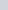). See figure belowGaussian surface for a long thin charged wire of uniform charged density

From the symmetry, the electric field is everywhere radial the plane cutting the wire normally and it’s magnitude depends on the radial distance r.
From the knowledge of gauss law-Now solving further, we get-The charge enclosed in the cylinder,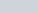The direction of the electric field is radially outward from the positive line charge but if the line charge is negatively charged then the electric field is radially inward.
Thus, the electric field (E) due to the linear charge is inversely proportional to the the distance (r). The variation of electric field (E) with distance (r) is shown in the figure below.

## ELECTRIC FIELD DUE TO THIN INFINITELY CHARGED PLANE SHEET

Let’s consider σ be the surface charge density of a plane charged sheet. From the symmetry, E will be either side of the sheet and must be perpendicular to the plane of the sheet. The electric field (E) will be same in magnitude at all the points equidistant from the plane sheet.

Take a cylinder of cross sectional area A and length 2r as Gaussian surface. See figure below:Gaussian surface for the infinitely thin charged plane sheet of surface charge density σ

On the curved surface of the cylinder the electric field (E) and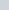are perpendicular to each other. Therefore, the electric flux through the curved surface is zero.
Flux through the flat surfaces is given as-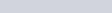Therefore, the total electric flux through the entire cylindrical surface is –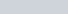Total electric field enclosed by the cylindrical surface is –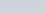According to the gauss’s law, we have –Here, you can see that, electric field is independent of r, the distance of the point from the plane charged sheet.
It means that electric field intensity remains same at all points close to the charged plane sheet. Electric field is directed away from the plane sheet if it is positively charged or if it is negatively charged then the electric field is directed inward.

## ELECTRIC FIELD DUE TO UNIFORMLY CHARGED THIN SPHERICAL SHELL

Let’s consider σ be the surface charge density of a thin spherical shell of radius R. The Gaussian surface is also a spherical surface of centre same as of the shell. See figure below:Gaussian surface for the uniformly charged spherical shell of density σ

### 1). At a point outside the shell, (r>R)

From the figure, E and dS are in the same direction. Therefore, electric flux is given as-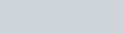Further solving, we get-Total electric charge on the spherical shell and further solution is-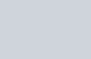Vectorially,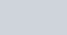### 2). At a point on the surface of the shell (r=R)

If the point is on the surface of the shell, then radius of the Gaussian surface and the radius of the shell is equal, (r = R), then the value of electric field is-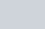### 3). At the point inside the shell,(r<R)

If the point is inside the shell, then there is no charge enclosed by the Gaussian surface. You can see in the above figure. It means q = 0, and this give, E = 0.
The variation of electric field intensity with distance from the centre of uniformly charged spherical shell is shown in figure below.